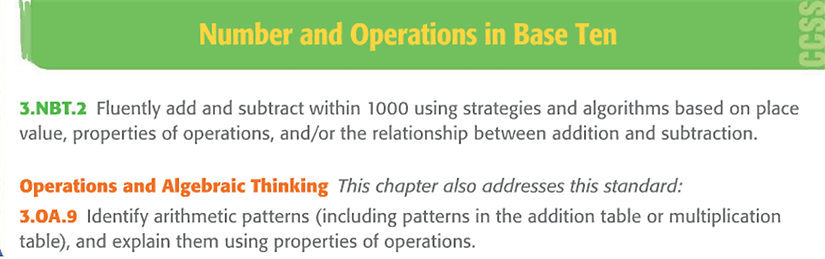top of pageVocabulary:

Associative Property
The property which states that the grouping of addends does not change the sum

Bar Diagram
A problem-solving strategy in which bar models are used to visually organize the facts in a problem

The property that states that the order in which two numbers are added does not change the sum5.
(4+5) + 2 = 4 + (5 +2)

Estimate
A number close to an exact value.  An estimate indicates about how much.
47 + 22 is about 70.

If you add zero to a number, the sum is the same as the given number.
3 + 0 =3 or 0 + 3 = 3

Mental Math
Ordering or grouping numbers so that they are easier to compute in your head.

Parentheses
Symbols that are used to groups numbers.  They show which operations to complete first in a number sentence

Pattern
A sequence of numbers, figures, or symbols that follow a rule or design.
2, 4, 6, 8, 10

Reasonable
Within the bounds of making sense.

Regroup
To use place value to exchange equal amounts when renaming a number.

Unknown
A missing number, or the number to be solved for.bottom of page